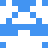### Real Vim ninjas count every keystroke - do you?

###### Pick a challenge, fire up Vim, and show us what you got.

```Your VimGolf key: please sign in

\$ gem install vimgolf
\$ vimgolf setup
\$ vimgolf put 52bc9404cbab90000200001c
```

### O Christmas Tree

Design a macro that makes trees grow (or ASCII triangles, if that's too hard).

```^

```
```^

^
^^^

^
^^^
^^^^^

^
^^^
^^^^^
^^^^^^^

^
^^^
^^^^^
^^^^^^^
^^^^^^^^^

```

#### View Diff

```2a3,20
>  ^
> ^^^
>
>   ^
>  ^^^
> ^^^^^
>
>    ^
>   ^^^
>  ^^^^^
> ^^^^^^^
>
>     ^
>    ^^^
>   ^^^^^
>  ^^^^^^^
> ^^^^^^^^^
>
```